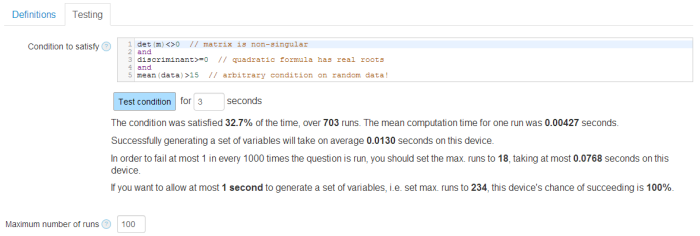# New tools to test generated variables

One thing that many people have asked for over the years is the ability to check whether the variables generated by a question have a desired property, and “re-roll” them if not. I’ve been reluctant to implement this because it can lead to sloppy question design when a method that’s guaranteed to work can often be found with a little bit of thinking.

The classic example of this is a question which asks the student to solve a quadratic equation $x^2+bx+c = 0$. You want to make the student’s life easier, so you want the equation to have real roots. The naive way of doing this is to pick random values for $b$ and $c$ until the discriminant $b^2-4c$ is non-negative. Since this is a random process, you can’t put an upper bound on how many tries it’ll take before a usable set of values is found.

The cleverer way to set up the question is to work backwards, by picking the roots $r_1$ and $r_2$ at random, and then expanding $(x-r_1)(x-r_2)$ to get $a$ and $b$. This process is deterministic, so there’s no need to re-roll.

However, you can’t always do this easily. One example that came up recently was a question which asked for a random nonsingular matrix. You can’t easily work back from a determinant to a whole matrix, and a random matrix is probably nonsingular, so it makes much more sense to just generate random matrices until you get a nonsingular one, and you can be fairly sure it’ll only take a few tries.

There’s another reason you might want to repeatedly regenerate a question’s variables: in a complicated question, it can be hard to work out if, and how often, pathological cases can come up. The way we used to check for this was to hit the “regenerate variables” button a few times, looking out for bad values. If nothing bad turned up after a minute or so of clicking, we were happy. Really, that should be automated.

With both those uses in mind, I’ve added a new “Testing” tab to the variable definition section of the Numbas question editor. You can write an expression (using the question’s variables) which should evaluate to true if the generated values are acceptable, and false otherwise. When a student runs the question, the variables will be regenerated until this expression is satisfied. While you’re writing the question, there’s a button you can press to generate as many sets of variables as possible in a given amount of time, and you’ll get a statistical summary of how often it failed.

Here’s how it looks:I took some time to decide what stats would be useful in the summary. You’re told how often the condition is satisfied, which is probably the first thing you look for. Everything after that is designed to give you an idea of how long it will take to generate a working set of variables when the question is run. The student might be using a slower device than you, so you should take that into account when interpreting the statistics. If the number of runs required to have an expected failure rate of 1 in 1000 would take too long, you should change the question.

If each question is taking longer than 1 second to generate its variables, the student will start to notice, so the summary tells you how likely it is that the process will succeed in time. If it’s much less than 100%, you should change the question.

This is all available now – I’ve made an example question to demonstrate how to use it, and of course there’s some documentation.